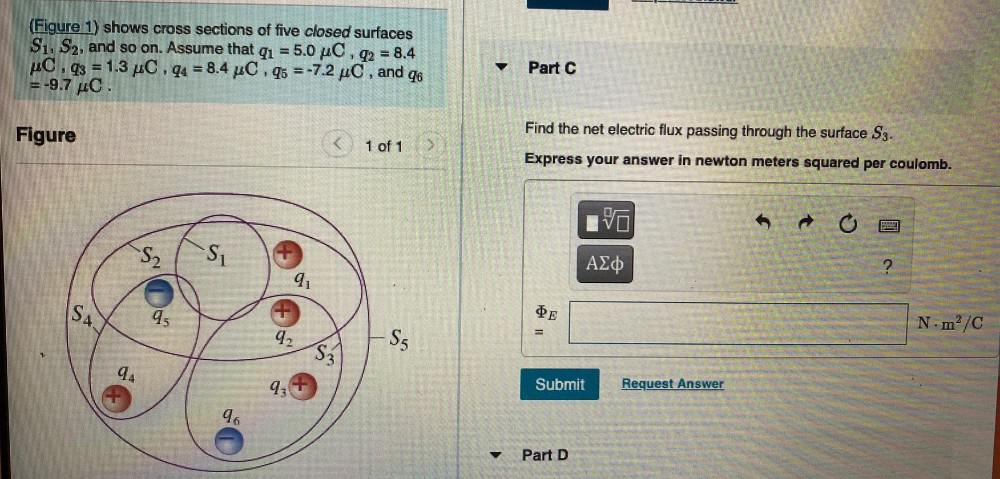Question:

# (Figure 1) shows cross sections of five closed surfaces S1, S2, and so on. Assume that qı = 5.0 pc, q2 = 8.4 C.q3 = 1.3 pc , q4(Figure 1) shows cross sections of five closed surfaces S1, S2, and so on. Assume that qı = 5.0 pc, q2 = 8.4 C.q3 = 1.3 pc , q4 = 8.4 pC.q5 =-7.2 pc, and 46 =-9.7 C. Part C Figure Find the net electric flux passing through the surface S3. 1 of 1 Express your answer in newton meters squared per coulomb. VO o S2 SU AZO 9 SA. 45 DE Nm²/C 42 Sg S3 94 93 Submit Request Answer 96 Part D